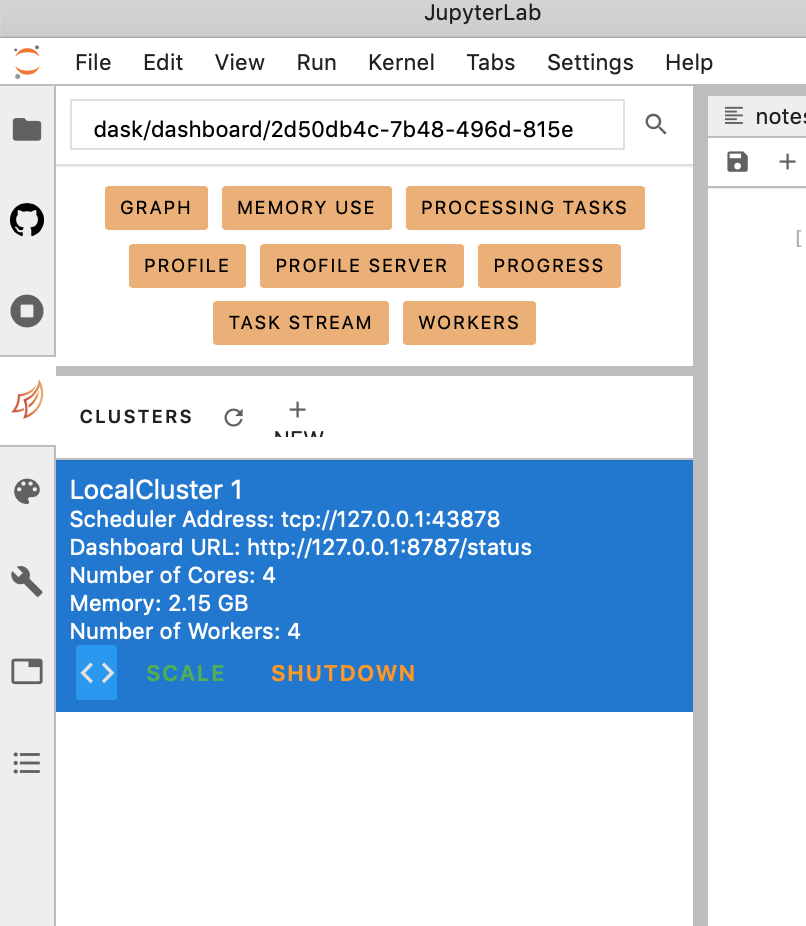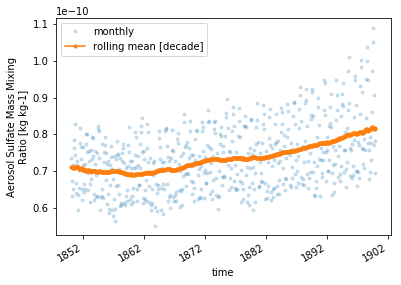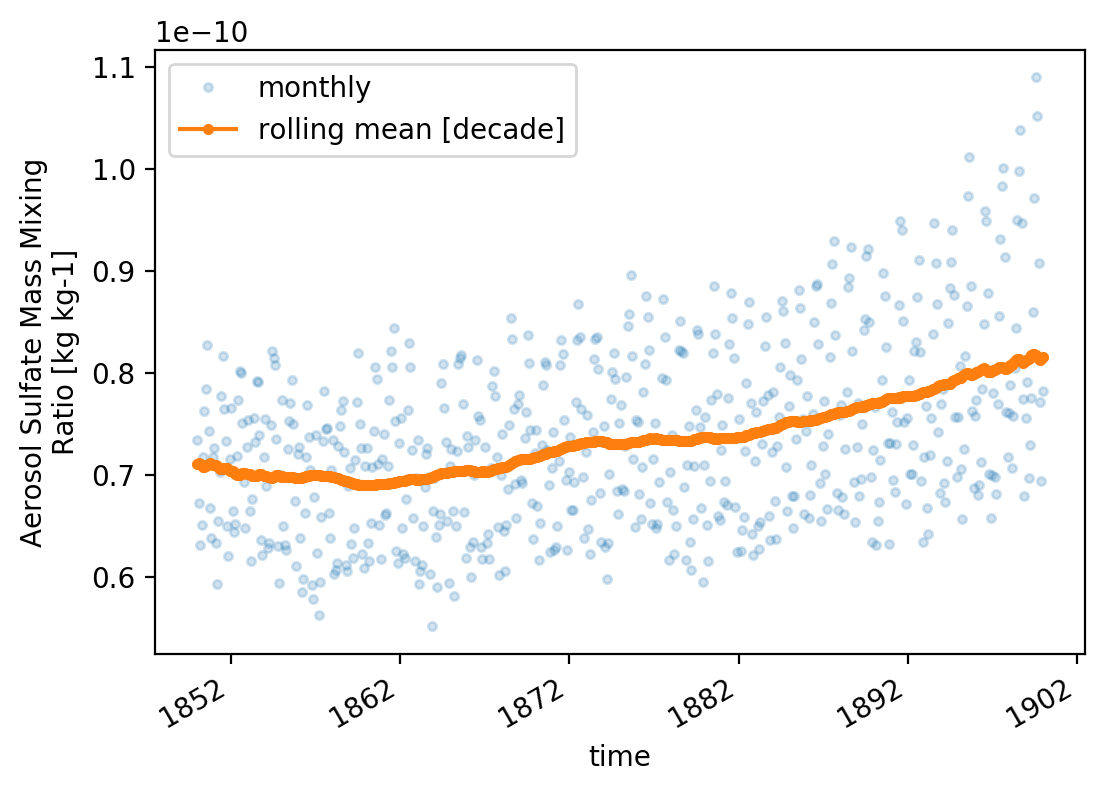from dask.distributed import Client

client = Client("tcp://127.0.0.1:38150")
client


### Cluster

• Workers: 4
• Cores: 4
• Memory: 2.15 GB

go to the dask array tab (shown below) and click on '<>'

from IPython.display import Imagesomething similar to the cell below should have being incerted

# imports

from negi_stuff.modules.imps import (
pd, np, xr, za, mpl, plt, sns, pjoin, os,
glob, dt, sys, ucp, log, crt, cmip6
)

from pathlib import Path


## select a large dataset

df = cmip6.search_cmip6_hist(wildcard='mmrso4*',model='CESM*')

_ds = df.sort_values(['FORCING','REALIZATION'],ascending=[False,True])

_ds = df.sort_values(
by       = [cmip6.FF,cmip6.RR,cmip6.FF],
ascending= [False   ,True    ,True]
)

_dg = _ds.groupby(cmip6.MODEL).first()

SIZE = 'SIZE'
df[SIZE] = df[cmip6.FILES].apply(lambda f: os.path.getsize(f))

_r = df.sort_values(SIZE, ascending=False).iloc

_r['FILE_PATH']

'/home/28f6ea40-2d3059-2d4f6b-2d8429-2deb8e956423db/shared-cmip6-for-ns1000k/historical/CESM2/r11i1p1f1/mmrso4_AERmon_CESM2_historical_r11i1p1f1_gn_185001-189912.nc'
large_file = '/home/28f6ea40-2d3059-2d4f6b-2d8429-2deb8e956423db/shared-cmip6-for-ns1000k/historical/CESM2/r11i1p1f1/mmrso4_AERmon_CESM2_historical_r11i1p1f1_gn_185001-189912.nc'


## open the xarray dataset

lets explore the dataset. however this is not opening the dataset as a [dask array] (https://examples.dask.org/xarray.html)

ds = xr.open_dataset(large_file)

/opt/conda/lib/python3.7/site-packages/xarray/conventions.py:494: SerializationWarning: variable 'mmrso4' has multiple fill values {1e+20, 1e+20}, decoding all values to NaN.
use_cftime=use_cftime,

#lets check the dimensions
ds.dims

Frozen(SortedKeysDict({'time': 600, 'lev': 32, 'lat': 192, 'lon': 288, 'nbnd': 2}))
#this will load the dataset as a dask array and therefore it will not kill the kernel
#some understanding of which operations you will perform on the dataset are needed
#in this case we are slicing the dataset along time in chunks of 1.
ds = xr.open_dataset(large_file,chunks={'time':1})

var = 'mmrso4'

# this will run fast but its just bc it hasn't been evaluated yet.
# so, daks will store the operations that you want on the dataset and only compute them
# when its necessary (e.g. when you want to plot the results). You can force the computation by
# calling .load() (see the next cell)
ds[var].mean()

<xarray.DataArray 'mmrso4' ()>
dask.array<mean_agg-aggregate, shape=(), dtype=float32, chunksize=(), chunktype=numpy.ndarray>
# in order to get the actual value you need to load
# this will take some time since now we are actually computing the mean.

ds[var][{'time':slice(0,None)}].mean().load()

<xarray.DataArray 'mmrso4' ()>
array(7.359101e-11, dtype=float32)
#convert cftime to datetime64
_c1 = ds['time'].item()
ds['time'] = pd.to_datetime(ds['time'].dt.strftime(_c1.format))

• first we average along the time dimension.
• then we load the results
• finally we copy the attrs for aesthaetics
da1 = ds[var].groupby('time').mean(xr.ALL_DIMS).load()
da1 = da1.assign_attrs(ds[var].attrs)

• we do a rolling mean.
da_rolling_mean = da1.rolling({'time':12*10},min_periods=1,center=True).mean()
da_rolling_mean = da_rolling_mean.assign_attrs(ds[var].attrs)

• define a function for plotting
def _plot():
ax = plt.axes()
l1 = 'monthly'

_plot()# increace res